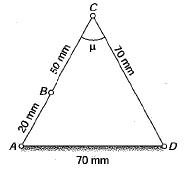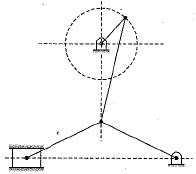Courses

# Test: Simple Mechanisms - 2

## 10 Questions MCQ Test Topicwise Question Bank for Mechanical Engineering | Test: Simple Mechanisms - 2

Description
This mock test of Test: Simple Mechanisms - 2 for Mechanical Engineering helps you for every Mechanical Engineering entrance exam. This contains 10 Multiple Choice Questions for Mechanical Engineering Test: Simple Mechanisms - 2 (mcq) to study with solutions a complete question bank. The solved questions answers in this Test: Simple Mechanisms - 2 quiz give you a good mix of easy questions and tough questions. Mechanical Engineering students definitely take this Test: Simple Mechanisms - 2 exercise for a better result in the exam. You can find other Test: Simple Mechanisms - 2 extra questions, long questions & short questions for Mechanical Engineering on EduRev as well by searching above.
QUESTION: 1

Solution:
QUESTION: 2

### Oldham’s coupling is used to connect two shafts which are

Solution:

Two shafts are parallel with offset.

QUESTION: 3

### What is the transmission angle for the following mechanism?Solution:

The given configuration represents equilateral triangle, so the transmission angle is 600.

QUESTION: 4

The mechanism shown in the given figure represents.Solution:
QUESTION: 5

A point on a connecting link (excluding end points) of a double slider crank mechanism traces a

Solution:
QUESTION: 6

A Kinematic chain is known as mechanism when

Solution:

A kinematic chain is known as mechanism when one link is fixed and D.O.F. = 1

QUESTION: 7

Degree of freedom of super structure is

Solution:

When the degree of freedom of a kinematic chain is less than zero then it is known as super structure.

QUESTION: 8

Degree of freedom of structure is

Solution:

When the degree of freedom of a kinematic chain is zero then it is called structure.

QUESTION: 9

If N is the number of link in a mechanism then number of possible inversion is equal to

Solution:

If there are N number of links, then the number of possible inversion will be equal to the number of links in the mechanism.

QUESTION: 10

A Kinematic chain requires at least

Solution:

A kinematic chain requires at least 4 link and 4 turning pair.